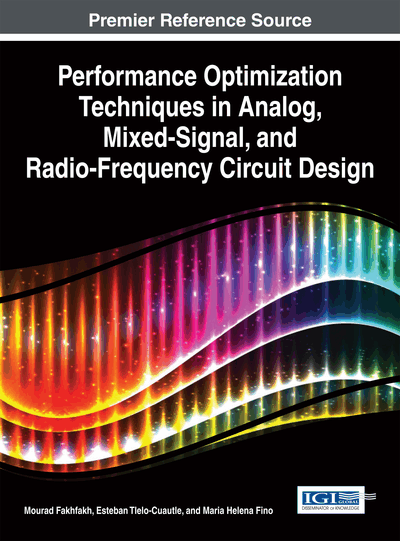# Thermometer to Gray Encoders

Yogendra Gupta (The LNM Institute of Information Technology, India) and Sandeep Saini (The LNM Institute of Information Technology, India)
Copyright: © 2015 |Pages: 13
DOI: 10.4018/978-1-4666-6627-6.ch013
OnDemand PDF Download:
Available
\$37.50
No Current Special Offers

## Abstract

Analog to Digital Converter (ADC) is a key functional block in the design of mixed signal, system on chip, and signal processing applications. An optimized method for the direct conversion of analog signal to Gray code representation is presented. This eliminates the need for binary-to-Gray code conversion in many digital modulation techniques like M-PSK and M-QAM, which uses Gray coding representation to represent the symbols that are modulated. The authors design a low-power and high-speed Thermometer to Gray encoder for Flash ADC, as encoders have been widely utilized in high-performance critical applications which persistently impose special design constraints in terms of high-frequency, low power consumption, and minimal area. In this chapter, they propose a new circuit that converts the Thermometer code to Gray code and also yields minimized power.
Chapter Preview
Top

## Introduction

In a communication system with real world data represent some physical quantity such as speech, temperature, etc. The real world physical quantity exists in the analog domain and if we want to send it via digital communication system, it is imperative to convert it into digital domain. So the process of converting an analog signal to digital signal is done by Analog to Digital Converter (ADC). The digital circuits are more robust and transmission errors are less and also digital circuit offers greater advantages over analog circuit in processing speed and efficient transmission of information. The current scenario of VLSI design for signal processing system demands high speed and low power consumption ADCs. In this chapter a method for direct conversion of analog signal to their Gray code representations is presented. This eliminates the need for binary-to-Gray code conversion in many digital modulation techniques. When multilevel signals are transmitted over a binary channel (PCM) or binary words transmitted over a multilevel channel, the mutual representation of the binary and non-binary signals are often in terms of Gray code, whose unit distance property proves to be of some advantage in such circumstances. So we have designed a low power and high speed Thermometer to Gray encoder, as encoders have been widely utilized in high-performance critical applications which persistently impose special design constraints in terms of high-frequency, low power consumption and minimal area.

Flash ADC is a type of ADC which offers highest sampling rate (Kanan, Kaess and Declercq, 1999). In the Flash ADC an array of comparators compares the input voltage with a set of increasing reference voltages. The comparator output represents the input signal in digital (Thermometer) code, which can be easily converted into Gray code.

Figure 1 shows the main structure of Flash ADC. In this figure there are three main parts. The First part is reference ladder network composed of a series of resistor. The reference ladder used to generateequally spaced reference voltages. In the second part an array of comparator compares the input voltage with the set of increasing reference voltages (Yoo Jincheol, Kyusun Choi, and Ali Tangel, 2001). If the input signal is greater than the reference voltage, the corresponding comparator output is high and denoted as logic “1”. On the other side, if the input signals voltage is lower than the specific reference voltage output is low and denoted as logic “0”. Note that comparison of the input signal with all reference voltage is performed at the same time.

As shown in figure the transition of output code from “1” to “0” denote the input signal voltage level because the reference voltage are arranged increasingly from the bottom to the top of comparators array, the output code will all be equal to “1” as the reference voltage is lower than input voltage level and all “0” as reference voltage is higher than input voltage level (Pereira & Fernandes, 2001). Hence all the comparator’s output looks like a Thermometer. Finally the third part is Thermometer to Gray encoder.

Gray coding representation that is used ubiquitously in all applications. In Gray coding, the adjacent representations (symbols) differ by only one bit. Gray coding, when combined with Forward Error Correction codes capable of corrective single bit errors, it can aid in correction of erroneous reception of bits that spills into adjacent symbols. Digital modulation techniques like M-PSK and M-QAM use Gray coding representation to represent the symbols that are modulated. In M-PSK and M-QAM modulation techniques if the constellation symbols are Gray encoded, and then the adjacent constellation symbol differs only by one bit. Thus this Gray encoded structure gives a lesser probability of error than natural binary ordering. Gray code is useful because only one bit changes at a time. When used as encoders for a position sensor, for instance, if the sensor were right at the edge of a change boundary, there is uncertainty.

## Complete Chapter List

Search this Book:
Reset